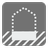Number Base
Simple
ES FR HU IT JA RU UK Simplified Chinese EN DE```checkio("AF", 16) == 175
checkio("101", 2) == 5
checkio("101", 5) == 26
checkio("Z", 36) == 35
checkio("AB", 10) == -1
```

re.match("\A[A-Z0-9]\Z", str_number)
0 < len(str_number) ≤ 10